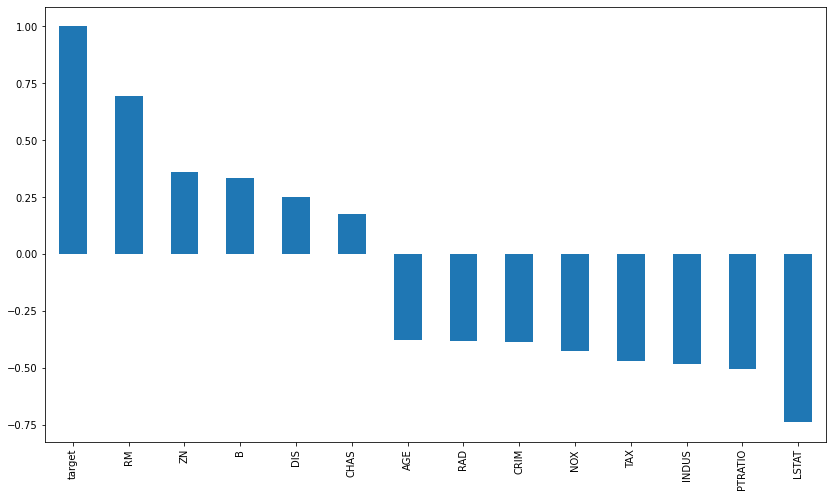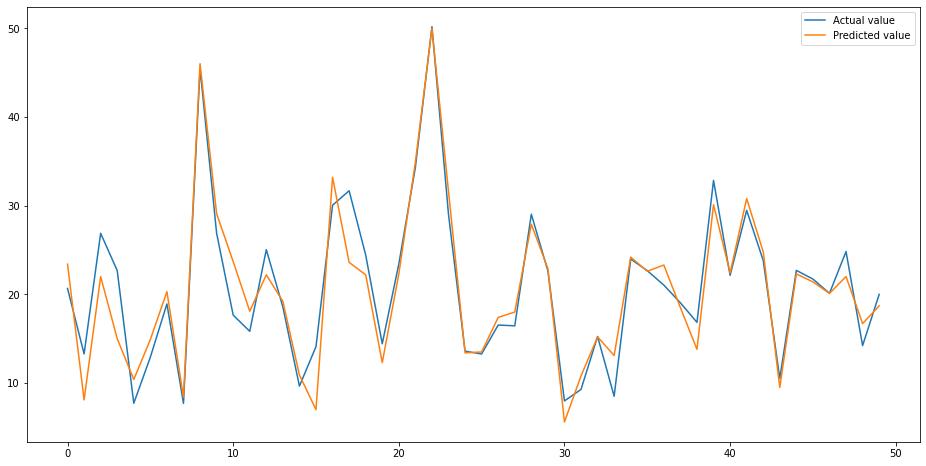Picture by Max Vakhtboych, Pexels.

One interesting technique in feature engineering is the use of Decision Trees (and other models) to create or derive new features using combinations of features from the original dataset. Here, small groups of features are selected from the columns in your training dataset and are used to train a sub-model, usually a Decision Tree. The predictions from the sub-model are then assigned back to the main dataset and used as features to help improve the performance of the main model.

This technique first came to light back in 2009 as part of the Knowledge Discovery in Data Competition (or KDD Cup 2009). It works in many situations, and doesn’t need to use a Decision Tree. However, it’s generally considered to be most effective when used to derive features that are “monotonic” with the target variable. Basically, a monotonic relationship means that as one of the variables increases, so does the other variable.

In this project, I’ll show you a simple example of how to apply the technique of using Decision Trees to derive new features from combinations from your original dataset, and show how it can help you improve the performance of your model.

For this simple example we only require a small selection of Python packages. Open a Jupyter notebook and import the packages below. We’ll be using the usual Pandas, Numpy, and Matplotlib packages to load and analyse our data, plus some packages from scikit-learn to split the training and test data, run a grid search, fit the Decision Tree model, and use its features in an XGBoost regression model.

``````import pandas as pd
import numpy as np
import matplotlib.pyplot as plt
from sklearn.model_selection import train_test_split
from sklearn.model_selection import GridSearchCV
from sklearn.tree import DecisionTreeRegressor
from xgboost import XGBRegressor
from sklearn import metrics
``````

To save the hassle of doing loads of data cleansing to prepare our data for use within the model, we’re going to use one of the example datasets built into scikit-learn. The dataset we’re using is the Boston House Prices dataset, which is designed for regression modeling. We’ll load the data, then convert it to a Pandas dataframe, making sure we include the `target` column containing the `y` variable we want the model to predict.

``````from sklearn.datasets import load_boston
``````
``````df = pd.DataFrame(data.data, columns=data.feature_names)
df['target'] = data.target
``````
CRIM ZN INDUS CHAS NOX RM AGE DIS RAD TAX PTRATIO B LSTAT target
0 0.00632 18.0 2.31 0.0 0.538 6.575 65.2 4.0900 1.0 296.0 15.3 396.90 4.98 24.0
1 0.02731 0.0 7.07 0.0 0.469 6.421 78.9 4.9671 2.0 242.0 17.8 396.90 9.14 21.6
2 0.02729 0.0 7.07 0.0 0.469 7.185 61.1 4.9671 2.0 242.0 17.8 392.83 4.03 34.7
3 0.03237 0.0 2.18 0.0 0.458 6.998 45.8 6.0622 3.0 222.0 18.7 394.63 2.94 33.4
4 0.06905 0.0 2.18 0.0 0.458 7.147 54.2 6.0622 3.0 222.0 18.7 396.90 5.33 36.2

Next, we’ll use Matplotlib to plot a bar chart of values from a Pandas Pearson correlation of the variables compared to the `target` column. Some values are positively correlated, while others are negatively correlated.

``````plt.figure(figsize=(14,8))
bars = df.corr()['target'].sort_values(ascending=False).plot(kind='bar')
``````### Split into test and training data

Now we’ll prepare the Pandas dataframe of features for use in our model. We’ll include all the feature columns in `X` by using the `drop()` function to remove the `target` (which would reveal the answer to our model). Then we’ll assign the `target` to `y`. We’ll use the scikit-learn `train_test_split()` function to randomly allocate the rows to either the training or test data, assigning 30% of the data to the test or validation dataset.

``````X = df.drop(columns=['target'])
y = df['target']

X_train, X_test, y_train, y_test = train_test_split(X, y, test_size=0.3, random_state=11)
``````
``````X_train.head()
``````
CRIM ZN INDUS CHAS NOX RM AGE DIS RAD TAX PTRATIO B LSTAT
255 0.03548 80.0 3.64 0.0 0.392 5.876 19.1 9.2203 1.0 315.0 16.4 395.18 9.25
49 0.21977 0.0 6.91 0.0 0.448 5.602 62.0 6.0877 3.0 233.0 17.9 396.90 16.20
124 0.09849 0.0 25.65 0.0 0.581 5.879 95.8 2.0063 2.0 188.0 19.1 379.38 17.58
503 0.06076 0.0 11.93 0.0 0.573 6.976 91.0 2.1675 1.0 273.0 21.0 396.90 5.64
11 0.11747 12.5 7.87 0.0 0.524 6.009 82.9 6.2267 5.0 311.0 15.2 396.90 13.27
``````y_train.head()
``````
``````255    20.9
49     19.4
124    18.8
503    23.9
11     18.9
Name: target, dtype: float64
``````

### Fit a base model

To see what sort of performance we get from a base model, we’ll fit a very basic XGBoost XGBRegressor model to the training data, then plot the predicted versus the actual house prices using some Matplotlib. As you can see, it does a decent job with barely any extra help from us.

``````regressor = XGBRegressor()
model = regressor.fit(X_train, y_train)
y_pred = model.predict(X_test)
``````
``````test = pd.DataFrame({'Predicted value':y_pred, 'Actual value':y_test})
fig= plt.figure(figsize=(16,8))
test = test.reset_index()
test = test.drop(['index'],axis=1)
plt.plot(test[:50])
plt.legend(['Actual value','Predicted value'])
``````
``````<matplotlib.legend.Legend at 0x7f98926ede50>
``````Next, we’ll assess the performance of our base model using a few different metrics from scikit-learn. We get a Root Mean Squared Error (RMSE) of 3.76, so are looking to reduce this score by creating a new feature and adding it back to the base model in the subsequent steps.

``````print('Mean Absolute Error (MAE):', metrics.mean_absolute_error(y_test, y_pred))
print('Mean Squared Error (MSE):', metrics.mean_squared_error(y_test, y_pred))
print('Root Mean Squared Error (RMSE):', np.sqrt(metrics.mean_squared_error(y_test, y_pred)))
``````
``````Mean Absolute Error (MAE): 2.404904535569643
Mean Squared Error (MSE): 14.172494681450521
Root Mean Squared Error (RMSE): 3.7646373904335757
``````

### Deriving a new feature using a Decision Tree

Now we’ve got our base model sorted, we can move onto deriving new features from the original data. To do this we’ll use GridSearchCV so we can optimise the `max_depth` parameter. There are loads of parameters (or hyperparameters) you can optimise within your model, but we’ll keep things simple for now, as adding more parameters slows things down, especially if you’re using a CPU-based model.

We’ll create a `DecisionTreeRegressor()` model and will use a 10-fold cross validation technique and assess its performance using the `neg_mean_squared_error` metric. In the next step, we’ll train the model and it will use 10 different splits on the data and try each of the max_depth values.

``````param_grid = {
'max_depth': [None, 2, 3, 4]
}

model = GridSearchCV(
DecisionTreeRegressor(random_state=11),
cv=10,
scoring='neg_mean_squared_error',
param_grid=param_grid
)
``````

Now we’ll create a list of features we want to combine within our Decision Tree model. I’ve picked three random ones, but you’ll want to play around with these and try different combinations (perhaps via Itertools) to see what works best for you. We’ll pass in the `selected_features` list to `X_train` so it only uses these three features to `fit()` or train the model.

``````selected_features = ['NOX', 'CRIM', 'ZN']
model.fit(X_train[selected_features], y_train)
``````
``````GridSearchCV(cv=10, estimator=DecisionTreeRegressor(random_state=11),
param_grid={'max_depth': [None, 2, 3, 4]},
scoring='neg_mean_squared_error')
``````

Once that’s done we’ll then use the `predict()` function to predict the target variable using the model training on our three selected values, and we’ll assign the prediction to a column called `NOX_CRIM_ZN` and assign it back to both the `X_train` and `X_test` data to ensure it’s present in both.

``````X_train = X_train.assign(NOX_CRIM_ZN=model.predict(X_train[selected_features]))
X_test = X_test.assign(NOX_CRIM_ZN=model.predict(X_test[selected_features]))
``````

If you print the `head()` of the `X_train` dataframe, you’ll now see the new feature we’ve created from the Decision Tree we trained to predict the median house value target variable using the three selected features. We’ve successfully created a new feature from our original list.

``````X_train.head()
``````
CRIM ZN INDUS CHAS NOX RM AGE DIS RAD TAX PTRATIO B LSTAT NOX_CRIM_ZN
255 0.03548 80.0 3.64 0.0 0.392 5.876 19.1 9.2203 1.0 315.0 16.4 395.18 9.25 26.698710
49 0.21977 0.0 6.91 0.0 0.448 5.602 62.0 6.0877 3.0 233.0 17.9 396.90 16.20 26.698710
124 0.09849 0.0 25.65 0.0 0.581 5.879 95.8 2.0063 2.0 188.0 19.1 379.38 17.58 19.104494
503 0.06076 0.0 11.93 0.0 0.573 6.976 91.0 2.1675 1.0 273.0 21.0 396.90 5.64 19.104494
11 0.11747 12.5 7.87 0.0 0.524 6.009 82.9 6.2267 5.0 311.0 15.2 396.90 13.27 19.104494

### Re-fit the model with the additional feature

To see if the new feature worked, we can now re-fit our original base model to the new `X_train` data and use it to predict on the new `X_train` data. Re-running the performance metrics we used earlier, show that the single extra feature has successfully reduced the RMSE value from 3.76 to 3.70, indicating that we likely have a better model fit due to the new feature.

Of course, this is only a single feature, selected entirely at random. With a more sophisticated approach, you could create and test a wide variety of different feature combinations to see which ones added the most performance to your model, and then use a technique such as Recursive Feature Elimination to select only the best ones.

``````regressor = XGBRegressor()
model = regressor.fit(X_train, y_train)
y_pred = model.predict(X_test)
``````
``````print('Mean Absolute Error (MAE):', metrics.mean_absolute_error(y_test, y_pred))
print('Mean Squared Error (MSE):', metrics.mean_squared_error(y_test, y_pred))
print('Root Mean Squared Error (RMSE):', np.sqrt(metrics.mean_squared_error(y_test, y_pred)))
``````
``````Mean Absolute Error (MAE): 2.4791884836397675
Mean Squared Error (MSE): 13.761760094911175
Root Mean Squared Error (RMSE): 3.709684635506255
``````
``````test = pd.DataFrame({'Predicted value':y_pred, 'Actual value':y_test})
fig= plt.figure(figsize=(16,8))
test = test.reset_index()
test = test.drop(['index'],axis=1)
plt.plot(test[:50])
plt.legend(['Actual value','Predicted value'])
``````
``````<matplotlib.legend.Legend at 0x7f98926edd90>
``````Matt Clarke, Sunday, June 20, 2021

Matt Clarke Matt is an Ecommerce and Marketing Director who uses data science to help in his work. Matt has a Master's degree in Internet Retailing (plus two other Master's degrees in different fields) and specialises in the technical side of ecommerce and marketing.